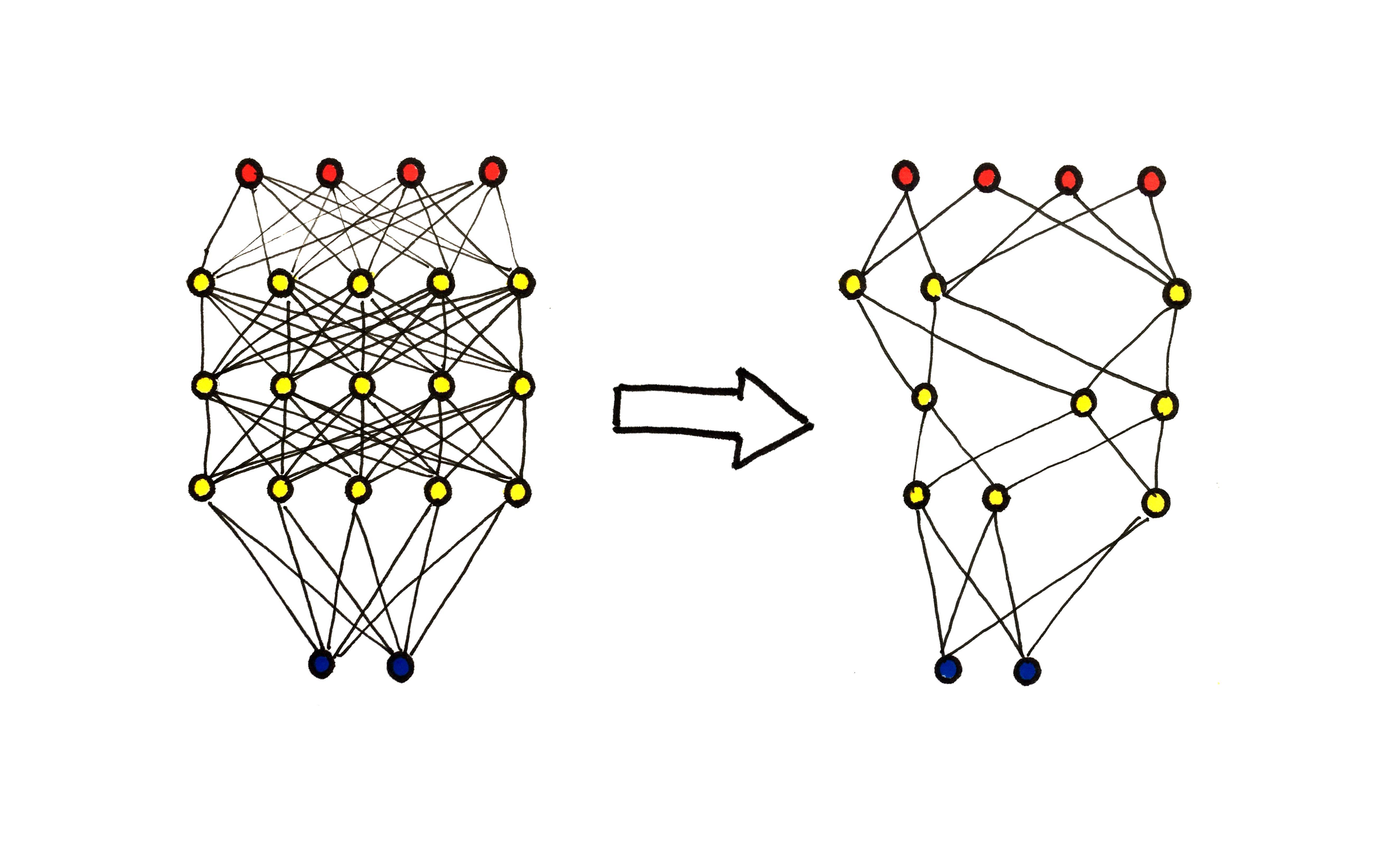# How to compress a neural network# How to compress a neural network

## An introduction to weight pruning, quantization, and knowledge distillation

Modern state-of-the-art neural network architectures are HUGE. For instance, you have probably heard about GPT-3, OpenAI’s newest revolutionary NLP model, capable of writing poetry and interactive storytelling.

Well, GPT-3 has around 175 billion parameters.

To give you a perspective about how large this number is, consider the following. A \$100 bill is approximately 6.14 inches wide. If you start laying down the bills right next to each other, the line will stretch 169,586 miles. For comparison, Earth’s circumference is 24,901 miles, measured along the equator. So, it would take ~6.8 round trips until we ran out of the money.

Unfortunately, as opposed to money, more is sometimes not better when it comes to the number of parameters. Sure, more parameters seem to mean better results, but also more massive costs. According to the original paper, GPT-3 required 3.14E+23 flops of training time, and the computing cost itself is in the millions of dollars.

GPT-3 is so large that it cannot be easily moved to other machines. It is currently accessible through the OpenAI API, so you can’t just clone a GitHub repository and run it on your computer.

However, this is just the tip of the iceberg. Deploying much smaller models can also present a significant challenge for machine learning engineers. In practice, small and fast models are much better than cumbersome ones.

Because of this, researchers and engineers have put significant energy into compressing models. Out of these efforts, several methods have emerged to deal with the problem.

# The why and the how

If we revisit GPT-3 for a minute, we can see how the number of parameters and the training time influences the performance.

The trend seems clear: more parameters lead to better performance and higher computational costs. The latter not only impacts the training time but the server costs and the environmental effects as well. (Training large models can emit more CO2 than a car in its entire lifetime.) However, training is only the first part of the life cycle of a neural network. In the long run, inference costs take over.

To optimize these costs by compressing the models, three main methods have emerged:

• weight pruning,
• quantization,
• knowledge distillation.

In this article, my goal is to introduce you to these and give an overview of how they work.

Let’s get started!

# Weight pruning

One of the oldest methods for reducing a neural network’s size is weight pruning, eliminating specific connections between neurons. In practice, elimination means that the removed weight is replaced with zero.

At first glance, this idea might be surprising. Wouldn’t this eliminate the knowledge learned by the neural network?

Sure, removing all of the connections would undoubtedly result in losing all that is learned. On the other part of the spectrum, pruning only one connection probably wouldn’t mean any decrease in accuracy.

The question is, how much can you remove until the predictive performance starts to suffer?

## Optimal Brain Damage

The first ones to study this question were Yann LeCun, John S. Denker, and Sara A. Solla, in their paper Optimal Brain Damage from 1990. They have developed the following iterative method.

1. Train a network.
2. Estimate the importance of each weight by watching how the loss would change upon perturbing the weight. Smaller change means less importance. (This importance is called the saliency.)
3. Remove the weights with low importance.
4. Go back to Step 1. and retrain the network, permanently fixing the removed weights to zero.

During their experiments with pruning the LeNet for MNIST classification, they found that a significant portion of the weights can be removed without a noticeable increase in the loss.

However, retraining was necessary after the pruning. This proved to be quite tricky since a smaller model means a smaller capacity. Besides, as mentioned above, training amounts for a significant portion of the computational costs. This compression only helps in inference time.

Is there a method requiring less post-pruning training, but still reaching the unpruned model’s predictive performance?

## Lottery Ticket Hypothesis

One essential breakthrough was made in 2008 by researchers from MIT. In their paper titled The Lottery Ticket Hypothesis, Jonathan Frankle and Michael Carbin stated that in their hypothesis that

A randomly-initialized, dense neural network contains a subnetwork that is initialized such that — when trained in isolation — it can match the test accuracy of the original network after training for at most the same number of iterations.

Such subnetworks are called winning lottery tickets. To see why let’s consider that you buy 10¹⁰⁰⁰ lottery tickets. (This is more than the number of atoms in the observable universe, but we’ll let this one slide.) Because you have so many, there is a tiny probability that none of them are winners. This is similar to training a neural network, where we randomly initialize weights.

If this hypothesis is true, and such subnetworks can be found, training could be done much faster and cheaper, since a single iteration step would take less computation.

The question is, does the hypothesis hold, and if so, how can we find such subnetworks? The authors proposed the following iterative method.

1. Randomly initialize the network and store the initial weights for later reference.
2. Train the network for a given number of steps.
3. Remove a percentage of the weights with the lowest magnitude.
4. Restore the remaining weights to the value that was given during the first initialization.
5. Go to Step 2. and iterate the pruning.

On simple architectures trained on simple datasets, such that LeNet on MNIST, this method offered significant improvement, as shown in the figure below.